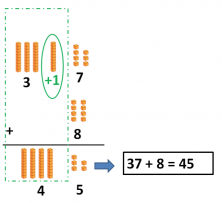Smartick is a fun way to learn math!Sep13

# Learn and Practice Vertical Adding

In today’s post, we are going to review vertical adding. As always, we are going to review with examples that help us understand what it consists of and how it’s done.

But before starting, let’s review the math cubes that we’re going to use in order to add. How do we represent a number with these math cubes? It’s easy! We’re going to use the single units as ones. They correspond to the number, for example: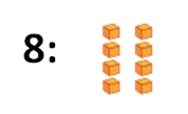And, so what do we need for the 10s? The 10’s bars make it easier to represent numbers so we don’t have to use lots of single units, instead, we can lump them together into a bar that has 10 units.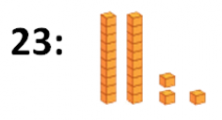We are going to start looking at some vertical adding exercises. To make sure we really understand it, we are going to solve three addition problems:

21 + 34

42 + 6

37 + 8

We are going to start with the first problem. Solving requires two steps: the first step consists of setting up the numbers that we’re going to add and the second step is actually adding the numbers together.

1. Setting up the Problem

In order to vertically add a problem, we need to make sure that we set the numbers up correctly. To do so, we need to line the number columns up properly. So, the 1s place lines up with the 1s place, the 10s place lines up with the 10s place, etc: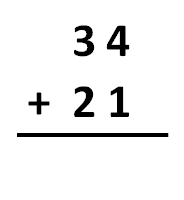To get a better idea, let’s use the math cubes: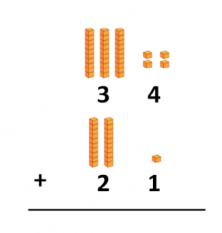Now, we just have to add, keeping two very important things in mind:

a) We are always going to add the numbers that fall in the same column.

b) We start with the 1’s column, and from there, we move on to the left.

So, first we add the 1’s column: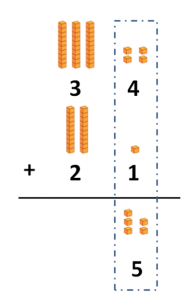And after, we add the 10’s column: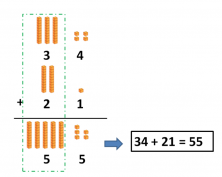And, what happens when there isn’t a number in a column? We do the same thing we did before: we line up the numbers in columns that go from right to left, and we add the columns, starting with the 1’s column. If there is only one number in a column, we don’t add anything to it.

#### Example 1

42 + 6

First, we add the 1s column: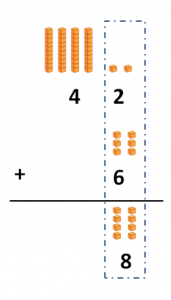And now, we move on to the 10s column.

The second number has a 0 in the 10s place, so the 10s place number stays the same: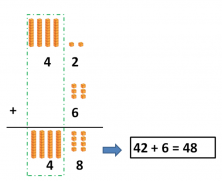And, what happens if when we add the numbers in a column, we get a number that’s greater than 9? Can we write 2 digits in the same column? Absolutely not! If this happens, we have to use regrouping.

#### Example 2

37 + 8

First, we add the 1s column. But 7 plus 8 gives us a 2 digit number. We can’t write two numbers in the same column, so we separate the sum 15. We put one of the digits in the 10’s column and one of them in the 1’s column.  In other words, we leave the 5 in the 1s place, and we carry over the 1 to the 10s place column: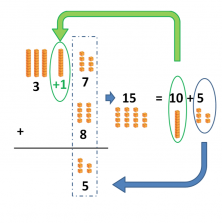Now we just need to add the 10s column.